Web of Science (IF=5.6, Q2) , ISC (Q1) , MSRT, CAS

Document Type : Original Article

Authors

1 Department of Chemistry, K. N. Toosi University of Technology, P. O. Box 16315-1618, Tehran 15418, Iran

2 Department of Chemistry, Space Transportation Research Institute, Iranian Space Research Center, Tehran, Iran

3 Research Group of Environmental Assessment and Risk, Research Center for Environment and Sustainable Development (RCESD), Department of Environment, Tehran, Iran

4 Department of Chemistry, Faculty of Science, University of Maragheh, PO Box 55181-83111, Maragheh, Iran

Abstract

In this research, the removal efficiency of an Acid Brown-14 as azo dye was carried out by an adsorbing method using a magnetic-activated carbon adsorbent, through which separation of adsorbent could be performed by a magnet at the end of the process. A second-order reduced polynomial model is used for method and optimization. Based on the model foresight, the process can remove the dye up to 82 % under the optimum situations of the primary pH = 4.6, [Dye] = 20 ppm, and [Ads.] = 791 ppm at room temperature. An experimentally accurate assessment of the model revealed that the model has a good agreement with the model prediction.

Graphical Abstract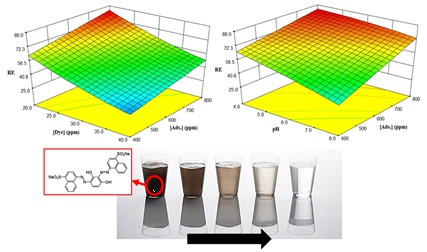Keywords

Main Subjects

###### ##### Full Text

Introduction

This work aims to remove a textile dye from an aqueous medium by a low-priced and useful adsorption method on magnetically activated carbon adsorbents. Activated carbon is simply separated from the water at the end of the work by using a magnet. In the light of the above concerns, the impact of parameters such as pH, adsorbent, primary dye molarity on the elimination efficiency of the dye has been studied and optimized.

Material and methods

Reagents and instruments

The azo dye of Acid Brown 14, C26H16N4Na2O8S2 with a molecular weight of 622.42 g/mol as a regular pollutant was purchased from AlvanSabet Company (Iran). FeCl3.6H2O, FeCl2.4H2O, and ammonia solution (25%) were bought to make adsorbents.  Sodium hydroxide and Sulfuric acid were used to set solution pH. All the substances were Merck and Fluka products. In each of the experiments, deionized water was used to prepare solutions. The concentration of the dye was measured by a UV–Vis spectrophotometer (Perkin Elmer Lambda 25). The structure of magnetic Nano-adsorbent was analyzed by Transmission Electron Microscope (TEM) (EM10C-100 KV).

Because of the superparamagnetic aspect of magnetite, it was selected for the magnetization of activated carbon . To prepare the magnetite nanoparticles, nitrogen gas was purged into 50 ml deionized water to deoxygenate it. Then, 1 g of activated carbon was added to the solution, and after well mixing, 0.79 g of FeCl3.6H2O and 0.29 g of FeCl2.4H2O were added and stirred again. Then, 3 mL of ammonia solution (25 wt. %) was added dropwise into the solution, and stirred continuously for 30 min. Notably, the solution was bubbled with nitrogen gas in all of the synthesis stages. Finally, the concerned magnetic activated carbon was washed several times using deionized water and ethanol and dried under vacuum at 60 ◦C for 5 h . TEM analysis was used to characterize synthesized magnetic activated carbon. The relevant image of the magnetic adsorbent has been depicted in Figure 1.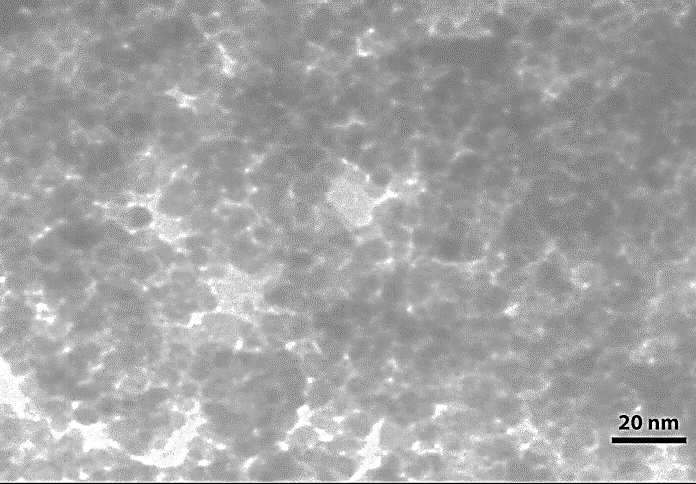Figure 1: TEM images of magnetic carbon Nano-composite

Procedure

To investigate the removal process of the dye by the adsorption process, a specific concentration of the dye solution was provided, and a certain amount of magnetic adsorbent was added. In each test, 50 ml of solution with a certain dye concentration were prepared, and then a specific amount of adsorbent was added to the solution. After a specific stirring time by the magnetic stirrer, the adsorbent was removed using a magnet. Then, to measure the effectiveness of the process and calculate the amount of contaminant removal, the residual concentration of dye in the solution was measured spectroscopically at a maximum wavelength of 455 nm. UV-Vis spectrum of Acid brown 14 dye has been demonstrated in Figure 2.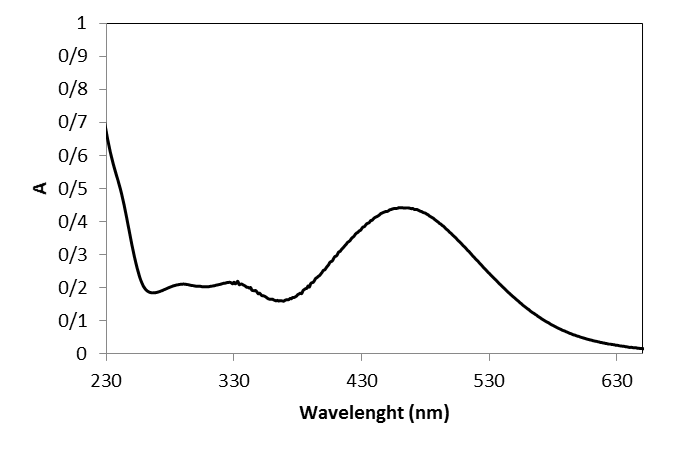Figure 2: UV-Vis spectrum of acid brown 14

Design of the experiments

Optimization means determining a specific value for the parameters affecting the system in which we have the best response. After determining the variables affecting the process, these variables must be optimized. The two most common methods used in laboratory studies to determine the optimal conditions are the classical method and statistical design. In the classical method, in addition to spending time and cost, the interferences are not known. Also in this method, the factors must be independent of each other. In recent years, researchers have considered the statistical design method for process optimization so that they can examine all aspects of the work by performing the least possible experiments and provide appropriate answers schematically and statistically through accurate calculations. One of the most effective methods in this field is the experimental design method. This method was first developed by Fisher and Yates in 1920 . To design and perform experiments, the most widely used method is the response level method. In recent years, in studies related to wastewater and water purification, this method has received much attention [16, 17].

Experiments designed by the CCD method consists of three points: Cubic, axial, and center points. The total number of experiments (N) designed by the method can be determined as follows :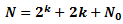(1)

where k, 2k, 2k, and N0 are the number of factors, the term of cubic points, the term of axial points, and the term of center points, respectively.

In this research, optimization of the three important factors of adsorbent dosage, solution pH, and initial dye concentration were performed with considering maximizing removal efficiency of the dye as the response factor by central composite design (CCD).

Result and Dissection

Experimental Design and statistical analysis

At this stage, according to the results of the initial tests and review of studies conducted in this field, the practical variables and their range were identified. For this purpose, the amount of adsorbent, the primary pH of the solution, and the primary concentration of the sample factors were chosen as operational effective variables, and the removal competence was selected as the response. Each parameter in this study was studied at five different levels with code values of −α, −1, 0, 1, + α. Table 1 shows the varieties and levels of the independent variable parameters used along with the CCD matrix as well as designed experiments and related experimental answers. To find the best model, after examining and evaluating the fit defects related to different models, a quadratic model was recommended by the software to explain the process. The proposed model was analyzed by study of variance (ANOVA), which is presented in Table 2. An F-value of 238.83 and a P-value of 0.0001 indicate that the proposed model is important for simulating the adsorption process of the dye by magnetic-activated carbon. The proposed model for the system includes three terms of single-component or linear effects (A, B, C), three terms of dual or interaction effects (AB, AC, BC), and three terms of quadratic effects (A2, B2, C2). However, not all of these parameters have a meaningful effect on the model and should be removed. Values less than 0.05 and greater than 0.1 for the P-value parameter belonging to each term of the model, respectively, indicate whether those terms are important or unimportant in the model .

In the recommended model, the "P-value" parameter is important for all expressions related to linear and quadratic terms, and interactive terms are irrelevant. Also, the F-value equal to 4.59 for the parameter Lack of Fit shows that the lack of fit is not notable. The sum of the squares R2 = 0.99 indicates that the model has great exactness. On the other side, the “Pred R-Squared” of 0.98 and “Adj R-Squared” of 0.97 represent the model predict the response as well. Also, the parameter of “Adeq Precision” indicates the signal-to-noise ratio, in which a ratio bigger than 4 is acceptable; in any case this parameter is equal to 53.97, which is a desirable value.

After statistical analysis, the proposed model was presented as a quadratic equation in terms of actual parameters by the software, which is shown in Equation (2). This mathematical equation presents the removal rate of the dye by magnetically activated carbon as a function of the value of various operating parameters.

Table 1: The range of variables and designed experiments by CCD with related experimental responses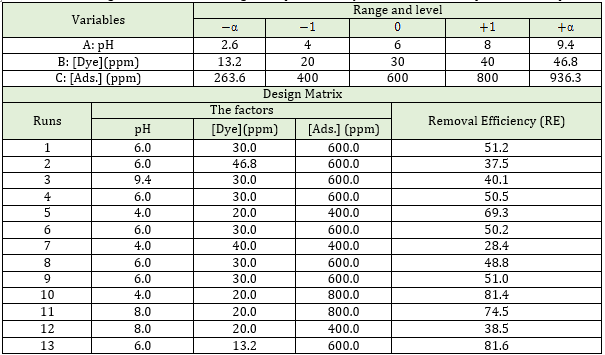Table 2: Analysis of variance for the response surface reduced quadratic model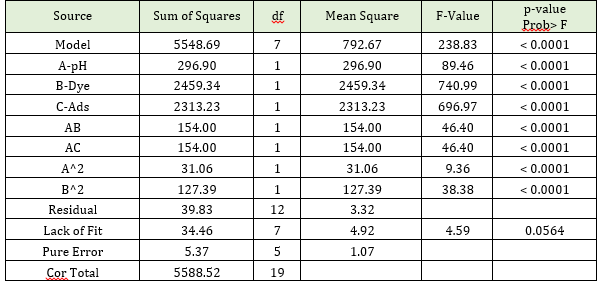Effect of operational parameters and process optimization

To study how each variable is affected and the interaction or dual effects of the variables on the response generated by the model, three-dimensional plots based on the polynomial function of the model were prepared using experimental design software. In these plots, the two variables change in the experimental range, while the other parameters are kept constant.

Figure 3 shows a three-dimensional diagram of the dye removal efficiency using magnetic-activated carbon as a function of the pH and amount of adsorbent dosage. It is observed that with increasing the amount of adsorbent and decreasing the pH, the removal efficiency increases. By decreasing the pH, the positive charge of the adsorbent surface increases, and its tendency to the dye increases. Also, with raising the amount of adsorbent, the number of active sites for the adsorption of pollutants increases, and as a result, the removal efficiency of dye increases.